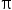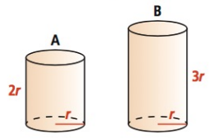Mathematics
Easy

Question

# An engineering firm wants to construct a cylindrical structure that will maximize the volume for a given surface area. The following formulas are given for the volume and surface area of cylinders.Volume (v) =r2hSurface Area (SA) = 2rh = 2r2calculate the ratio of volume for cylinder A to volume for cylinder B?

## 1 : 32 : 3 1 : 1 3 : 2Hint:

## The correct answer is: 2 : 3

### Related Questions to study#### With Turito Foundation.#### Get an Expert Advice From Turito.